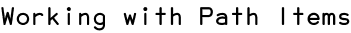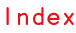RSS

In this tutorial we are going to look at paths and the different ways to create and modify them in Illustrator documents.

## The Anatomy of Path Items

In Illustrator, paths are represented by a sequence of segments that are connected by curves. A segment consists of a point and two handles, defining the location and direction of the curves.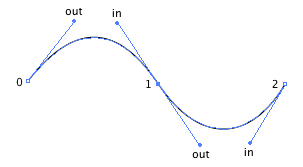In order to start with paths here, we will use segments that do not define handles for now and therefore are connected by lines instead of curves.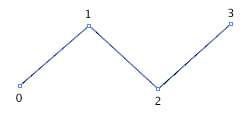Scriptographer helps us here by converting Point objects to Segment objects on the fly wherever necessary. So when we created an empty path in Writing Your First Script and added points to it using the path.add(segment) function, we in fact asked Scriptographer to convert these points to segments on the fly:

```var myPath = new Path();
```

In the Writing Your First Script tutorial we created an empty path by using the new Path() constructor and added segments to it using the path.add(segment) function:

```var myPath = new Path();
```

The add function also supports multiple arguments, which allows you to insert multiple segments in one go:

```var myPath = new Path();
myPath.add(new Point(0, 0), new Point(100, 50));
```

To insert segments in relation to already existing segments, you can use the path.insert(index, segment) function:

```var myPath = new Path();
myPath.add(new Point(0, 0), new Point(100, 50));

// insert a segment between the two existing
// segments in the path:
myPath.insert(1, new Point(30, 40));
```

The Point object represents a point in the two dimensional space of the Illustrator document. It does not refer to an anchor point in a path. When a Point is passed to a function like add or insert it is converted to a Segment on the fly. To find out more about the Point object, please read the Point, Size and Rectangle tutorial.

## Smoothing Paths

Scriptographer allows you to automatically smooth paths using the path.smooth() function. This function calculates the optimal values for the handles of the path's segment points to create curves that flow smoothly through them. The segments are not moved and the current handle settings of the path's segments are ignored.

In the following example, we create a path, add 4 points to it and then smooth it:

```var myPath = new Path();

myPath.smooth();
```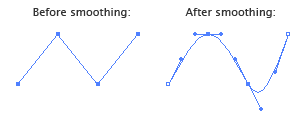## Closing Paths

By default paths created through the new Path() constructor are open:

```var myPath = new Path();
```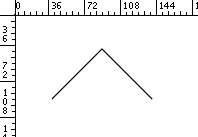To close the path we set its path.closed to true.

```myPath.closed = true;
```

Illustrator will then connect the first and last segments of the path: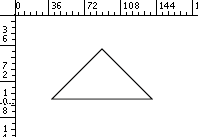## Removing Segments and Paths

To remove a segment from a path, we use the path.remove(index) function and pass it the index of the segment we want to remove.

If you don't know yet how to create paths of predefined shapes, please read the the Creating Predefined Shapes tutorial.

First, lets create a circle shaped path and inspect its segments:

```var myCircle = new Path.Circle(new Point(100, 100), 50);
```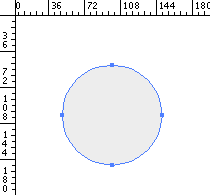As you can see, the path has 4 segments.

Now, lets remove the first segment of the path after creating it:

```var myCircle = new Path.Circle(new Point(100, 100), 50);
myCircle.remove(0);
```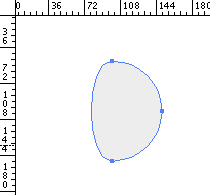Did you know?

In Javascript and most other programming languages we always start counting at zero when we refer to the index of an item in a list. Number zero is the first item, number one is the second item etc.

To remove a path completely from the document, we use path.remove() without passing it an index:

```var myCircle = new Path.Circle(new Point(100, 100), 50);
myCircle.remove();
```